# Dimension invariant

An integer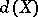, defined for every topological spaceof a given class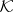, which has sufficiently many properties to make it resemble the usual notion of dimension: the number of coordinates of higher-dimensional Euclidean spaces. Here one requires of the classthat it contains all cubes with any number of coordinates, and together with any spacewhich is an element of it, it should also contain as an element every space homeomorphic to. For a dimension invariantit is assumed, in any case, that for homeomorphic spacesandone always has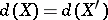, and that for the-dimensional cube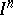one has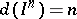. Among the dimension invariants, the most important ones are the so-called classical dimensions — the Lebesgue dimension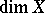and the (large and small) inductive dimensions (cf. Inductive dimension),.

The following propositions distinguishfrom all other dimension invariants defined, respectively, in the class of all (metric) compacta, all metrizable and all separable metrizable spaces, and hence settle for these spaces the problem of the axiomatic definition of dimension. The only dimension invariantsatisfying the conditions 1), 2), 3) listed below and defined in the classof all (metric) compactais the dimension(Aleksandrov's theorem).

Condition 1) (Poincaré's axiom). If a spaceis of classand ifis equal to the non-negative integer, thencontains a closed subspace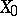for which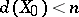and such that the set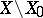is disconnected.

Condition 2) (the finite sum axiom). If a spaceof classis the union of two closed subspaces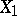and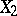for which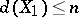,, then also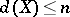.

Condition 3) (Brouwer's axiom in metric form). Ifis a space belonging toand ifis the non-negative integer, then there is a positive numbersuch that for every spacewhich is the image ofunder some-mapping one has the inequality. Here a mappingfrom a compactumonto a compactumis called an-mapping if it is continuous and if the complete pre-image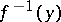of every point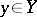has diameterin.

Shchepin's theorem . The dimensionis the only dimension invariantdefined, respectively, in the classof all metric, or all separable metric spaces, which satisfies the following conditions (Shchepin's theorem):

Condition 1) (Poincaré's axiom). See above.

Condition 2) (the countable sum axiom). If a spacebelonging to the classis the union of a countable number of closed subspaces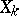,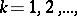each having, then also.

Condition 3) (Brouwer's axiom in general form). If for a spacebelonging to the classone has, then there is a finite open coveringofsuch that for every spacebelonging toand which is the image ofunder some-mapping one has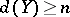. Here a mappingfrom a spaceon which a certain open coveringhas been fixed onto a spaceis called an-mapping if every pointofhas a neighbourhood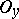whose complete pre-imageis contained in some element of.